# GED Science : Physics

## Example Questions

### Example Question #1 : Physics

Which of the following best illustrates Newton's Second Law?

A ball is rolled across a table and does not stop until it hits an area with friction

If an object is not accelerating, then the net force on it is zero

As a hammer hits a nail, the nail also exerts a force on the hammer

When two cymbals collide, some kinetic energy is converted into sound

If an object is not accelerating, then the net force on it is zero

Explanation:

Newton's Second Law is best represented as an equation, in which the product of mass and acceleration is equal to net force: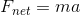By this principle, if there is zero acceleration, then the force must also be zero.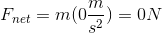A non-zero acceleration must be present if there is a non-zero net force.

### Example Question #1 : Physics

Which of the following is the best example of Newton's Third Law?

A spinning top will not fall unless there is friction with the surface on which it spins

If an object's weight rests on the floor, then the floor will exert a normal force on the object

When two objects collide, the total initial and total final momentum will be equal

The force of being hit by an object is determined by its mass and acceleration

If an object's weight rests on the floor, then the floor will exert a normal force on the object

Explanation:

Newton's Third Law states that for every action there is an equal and opposite reaction. In other words, a force will act in equal and opposite directions on the two objects involved.

Weight and normal force are a specific example of this principle. Acceleration due to gravity creates a downward force, known as weight. This force, however, results in zero downward acceleration when the object is resting on a surface (a book does not fall through a table, for example). For the forces to be in equilibrium, there must be a counteracting upward force. This is known as the normal force, which is equal and opposite to the weight of the object. While weight pulls the object down, normal force pushes it up to create equilibrium.

### Example Question #1 : Displacement

A car drivesmiles east andmiles north. What is the total displacement of the car?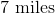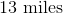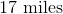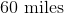Explanation:

The car has covered a total distance ofmiles, however, displacement and distance are not the same thing. Distance is simply the measure of how far the car have moved, regardless of direction. Displacement is a vector quantity, and takes direction into account. Displacement measures the total distance only between the car's starting point and ending point.

To solve this question, it is best to draw a sketch. The resulting sketch will be a right triangle with the hypotenuse equal to the displacement. The Pythagorean Theorem can be used to solve the problem.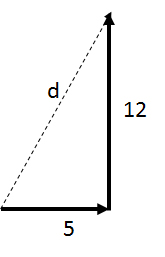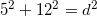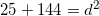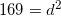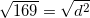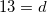### Example Question #1 : Ged Science

A car travels due east for two hours and covers 144km. What is the car's average velocity in meters per second?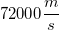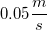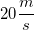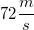Explanation:

This question requires us to convert between units, then solve for the velocity.

First, convert kilometers to meters. There are 1000m in 1km.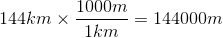Then, convert hours to seconds. There are 60min in 1hr, and 60sec in 1min. In other words, there are 3600s in 1hr.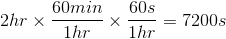Now that you have both the distance in meters and the time in seconds, you can find the velocity. Velocity is equal to the distance divided by the time.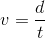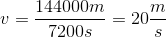### Example Question #1 : Kinetic Energy

A ball that has a mass of 2kg is launched upward at a velocity of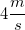. What is the initial kinetic energy of the ball?

Assume the ball is traveling in a vacuum.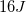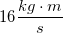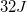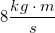Explanation:

Kinetic energy (measured in Joules) can be solved using the equation: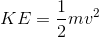We are given both the mass of the projectile (2kg) and its velocity (). Using these values, we can solve the equation.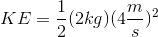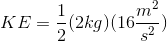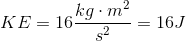### Example Question #1 : Physics

Which of the following measures depicts the amplitude?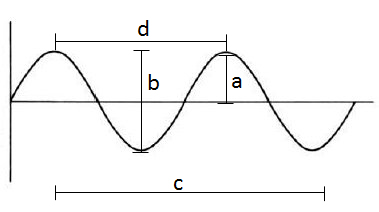A

D

B

C

A

Explanation:

The amplitude measures the greatest displacement (either positive or negative) of a wave from the x-axis. Visually, the amplitude appears to be the distance from the x-axis (center line) to the top of one peak. In the figure, this value is given by A.

The other relevant measure given in the figure is segment D, which measures the distance between two peaks. This distance is known as the wavelength.

Segments B and C do not provide useful measurements of the wave function.

### All GED Science Resources